﻿ 计及板厚腐蚀的半潜式平台疲劳裂纹扩展评估
«上一篇文章快速检索 高级检索

 哈尔滨工程大学学报2019, Vol. 40Issue (8): 1406-1413  DOI: 10.11990/jheu.2018071070

### 引用本文ZHAO Shilun, CHEN Chaohe, JIANG Yong, et al. Fatigue crack growth assessment of semisubmersibles based on plate thickness diminution[J]. Journal of Harbin Engineering University, 2019, 40(8), 1406-1413. DOI: 10.11990/jheu.201807107.### 文章历史

Fatigue crack growth assessment of semisubmersibles based on plate thickness diminution
ZHAO Shilun , CHEN Chaohe, JIANG Yong , SHEN Yijun
Naval Architecture and Ocean Engineering R & D Center of Guangdong Province, South China University of Technology, Guangzhou 510000, China
Abstract: This work focuses on the calculation and prediction of fatigue crack growth life. In this work, the fatigue assessment of existing cracks in the connection between the columns and braces of semisubmersibles is performed by considering plate thickness diminution. The appropriate coefficient of plate thickness diminution is selected in accordance with the code. Then, the exponential rate model is applied to calculate the remaining plate thickness after 20 years. The size of the remaining plate thickness is input to analyze the overall and local responses of the structure. The wave dispersion data and transfer function are used to obtain the discretized random fatigue load. Crack growth is calculated by applying a single curve model of crack growth rate. The failure assessment diagram (FAD) is added to the propagation cycle for safety evaluation. The calculated critical size and life of the crack are smaller than those of a plate with complete thickness, and the crack propagation life of a load with a single constant amplitude is smaller than that of a discrete random load. Results show that the rationality of the evaluation and calculation can be improved by accounting for plate thickness diminution in the analysis of the structural response of semisubmersibles and by using the discretized mode to address random fatigue load and FAD to evaluate crack growth.
Keywords: semisubmersible    crack growth    thickness diminution    fatigue analysis    failure assessment diagram (FAD)    fatigue crack propagation    growth rate    flaw assessment

1 疲劳扩展分析方法

1.1 疲劳载荷离散化处理

1) 针对不同工况，选取一系列浪向、波高与周期的组合，进行海洋工程结构物的整体有限元应力响应分析，计算其在单位波高下同一周期下不同相位的应力响应；

2) 提取同一周期下的最大应力σmx及最小应力σmn，计算传递函数的疲劳应力幅:

 $\Delta \sigma_{\text { unit }}=\operatorname{SCF}\left(\sigma_{m x}-\sigma_{m n}\right)$ (1)

3) 某工况下的疲劳扩展应力幅Δσi的大小可以利用波高Hi乘以传递函数的单位波高疲劳应力幅Δσunit:

 $\Delta \sigma_{i}=H_{i} \cdot \Delta \sigma_{\mathrm{unit}}$ (2)

 $n_{y i}=365 \times 24 \times 3600 \times p_{i} p_{j} / T_{z i}$ (3)

1.2 疲劳裂纹扩展率模型

 $\mathrm{d} a / \mathrm{d} N=A\left[\left(\Delta K_{R 0}\right)^{m}-\left(\Delta K_{R t h 0}\right)^{m}\right]$ (4)

 $\Delta K_{R 0}=M_{R} \Delta K$ (5)

1.3 疲劳扩展评估流程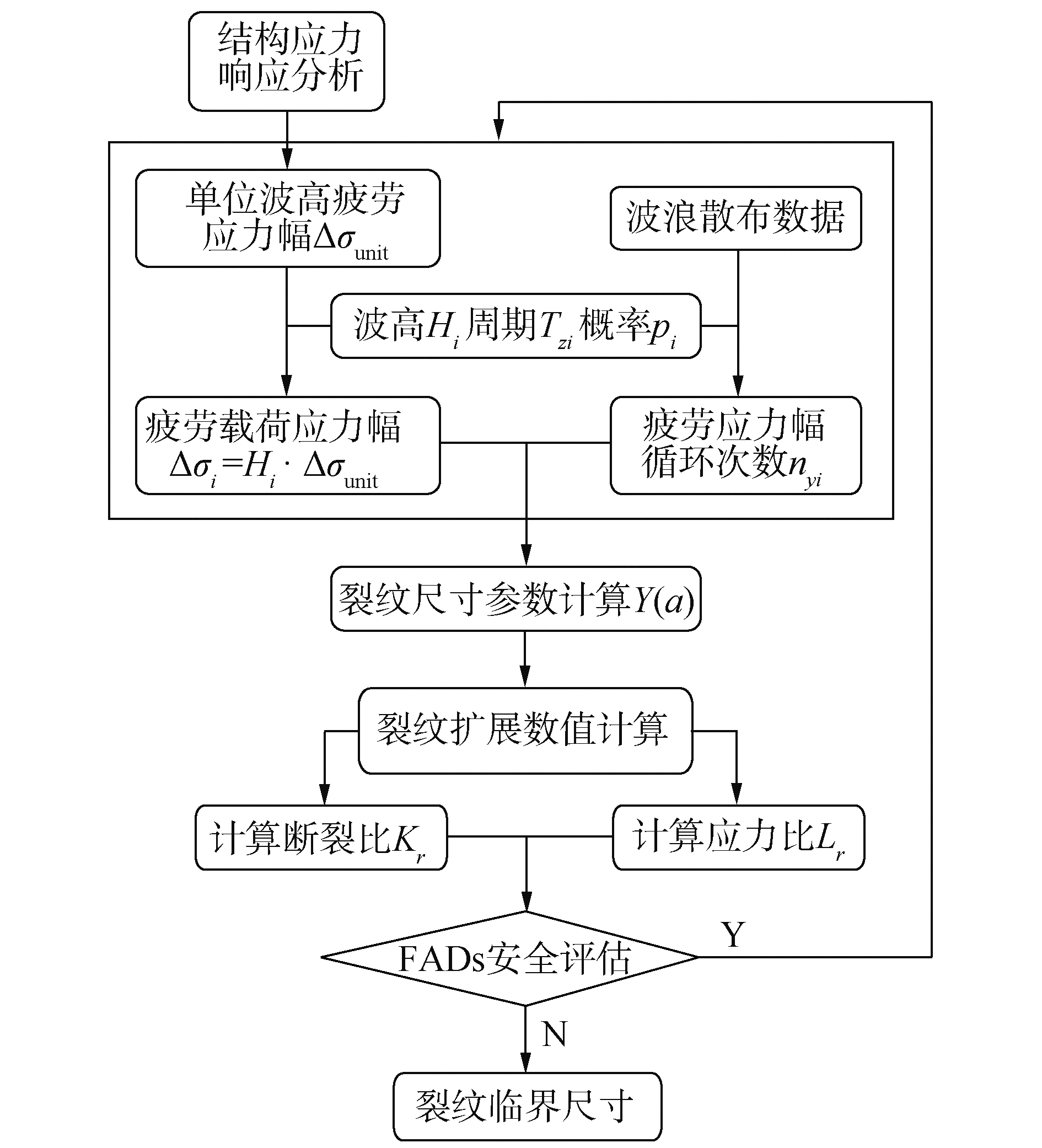Download: 图 1 疲劳评估流程 Fig. 1 Flowchart of fatigue assessment

1) 由于波浪载荷对于海洋工程结构物的作用是近似平衡随机的，因此进行疲劳载荷离化时忽略载荷加载次序的影响。

2) 疲劳扩展常数Am按照BS 7910的建议，可以选取单一裂纹扩展模型的参数，20 ℃海洋环境下，A=2.3×10-12m=3

3) 应力强度因子通过式(6)计算为：

 $\Delta K=Y(a) \cdot \Delta \sigma \sqrt{\pi a}$ (6)

4) 由于材料应力-应变数据的缺乏，因此本文将选用Option 1评估曲线进行裂纹扩展的安全计算。

Option 1评估曲线表达式为：

 $\left\{ \begin{array}{l} f\left( {{L_r}} \right) = {\left( {1 + 0.5L_r^2} \right)^{ - 0.5}} \cdot \left[ {0.3 + 0.7\exp \left( { - \mu L_r^6} \right)} \right],\\ \;\;\;\;\;\;\;\;\;\;\;\;\;\;\;\;\;\;\;\;\;\;\;\;\;\;\;\;\;\;\;\;{L_r} \le 1\\ f\left( {{L_r}} \right) = f(1) \cdot L_r^{(N - 1)/(2N)},1 < {L_r} \le {L_{r,\max }}\\ f\left( {{L_r}} \right) = 0,\;\;\;\;\;\;\;\;\;\;\;\;\;\;\;\;\;\;\;\;{L_r} > {L_{r,\max }} \end{array} \right.$ (7)

5) 生产厂商提供的结构物材料的断裂韧性数据为裂纹尖端张开位移δC，可通过式(8)获得断裂韧性KC

 $\delta_{C}=K_{C}^{2} /\left(X \sigma_{y} E^{\prime}\right)$ (8)

2 结构板厚削减的分析方法

2.1 板厚削减程度与发生区域

 $t_{c}=(1-k) t_{\mathrm{gross}}$ (9)表 1 平台区域对应板厚减小系数 Table 1 Diminution coefficients for structural component
2.2 板厚腐蚀速率模型

 ${d_p}\left( t \right) = \left\{ \begin{array}{l} 0,\;\;\;\;\;\;\;\;\;\;\;\;\;\;\;\;\;\;\;\;\;\;\;\;\;\;\;t \le {\tau _c}\\ {d_{p\infty }}\left( {1 - {{\rm{e}}^{ - \left( {t - {\tau _c}} \right)/{\tau _t}}}} \right),\;\;\;\;\;t > {\tau _c} \end{array} \right.$ (10)

 ${\tau _t} = {d_{p\infty }}/\tan \alpha$ (11)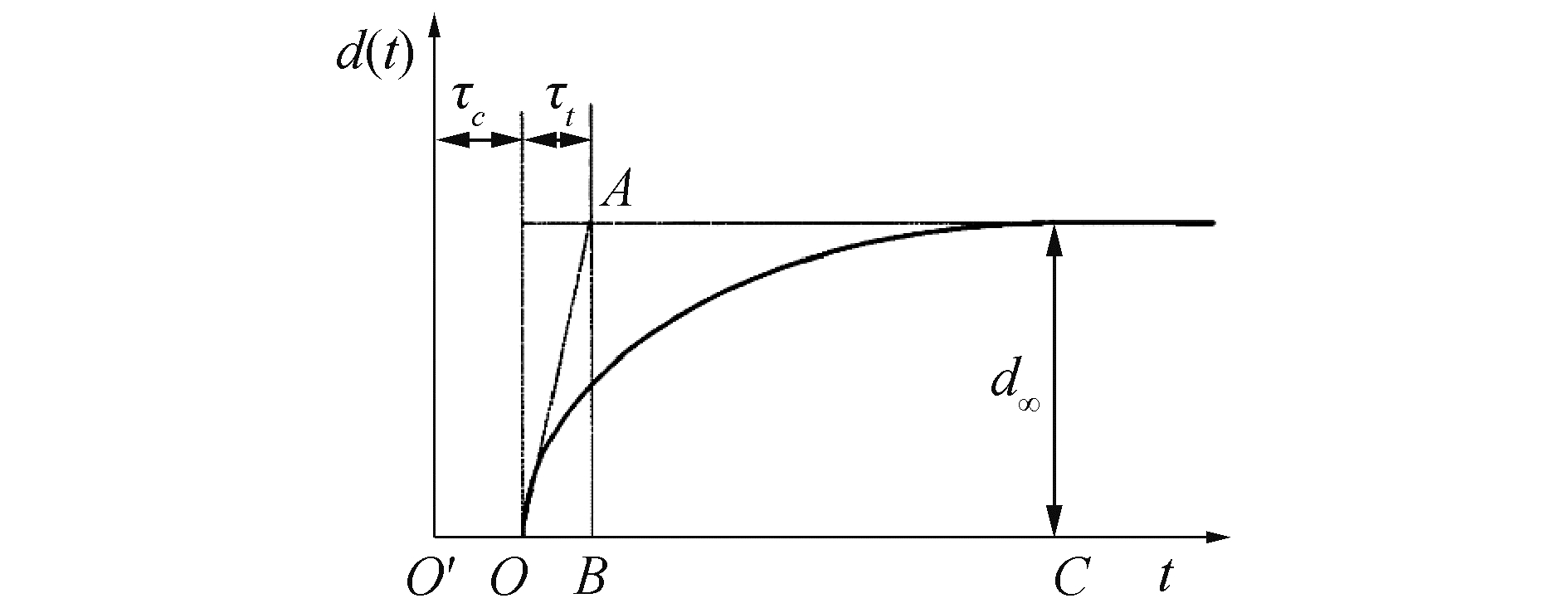Download: 图 2 板厚腐蚀随时间变化指数曲线 Fig. 2 Curve of plate corrosive thickness versus time
3 半潜式平台疲劳裂纹扩展评估 3.1 平台结构建模与裂纹基本信息表 2 半潜式平台主要尺寸参数 Table 2 Principle dimension of semisubmersible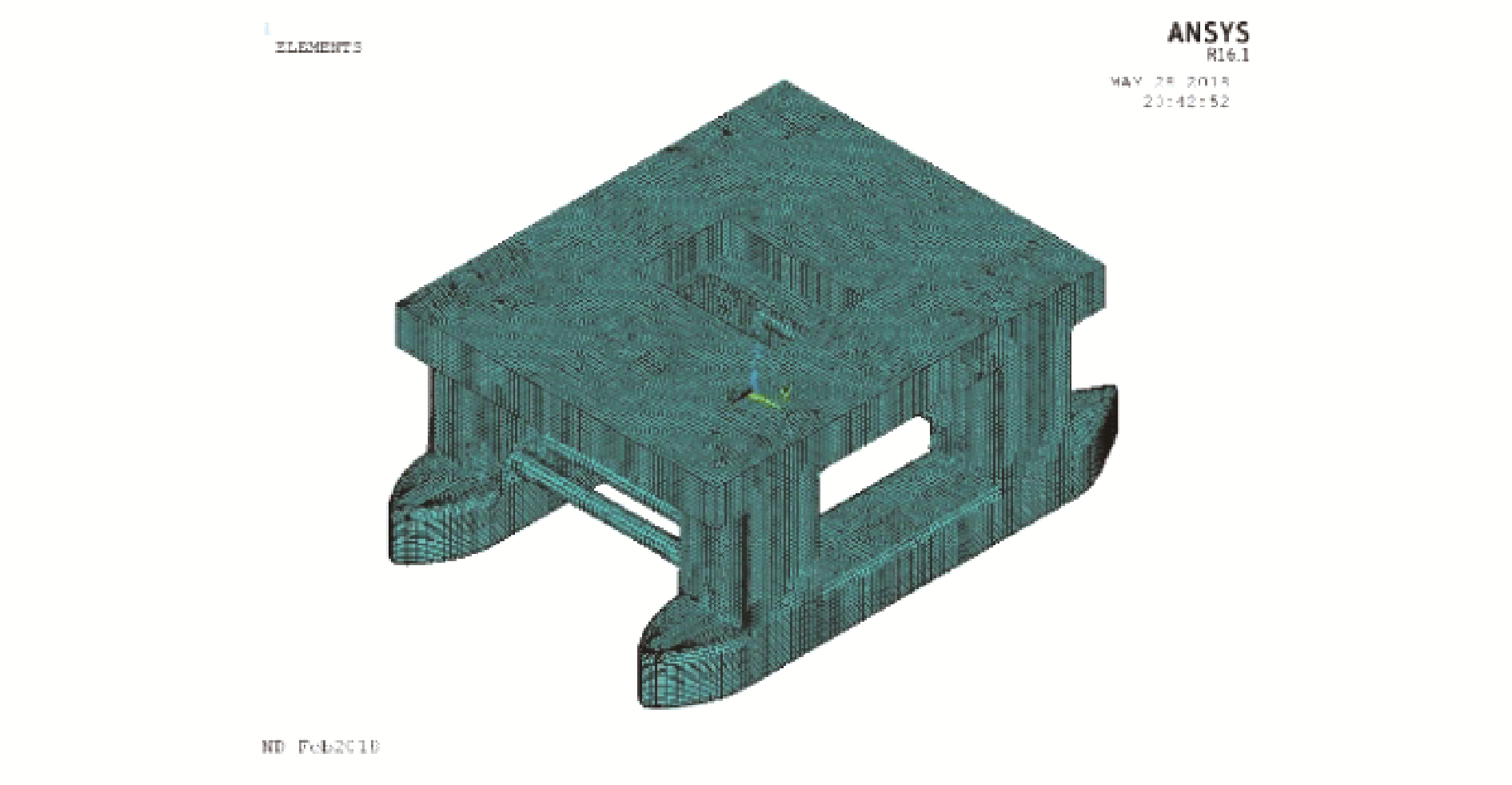Download: 图 3 平台整体结构有限元模型 Fig. 3 Global FE model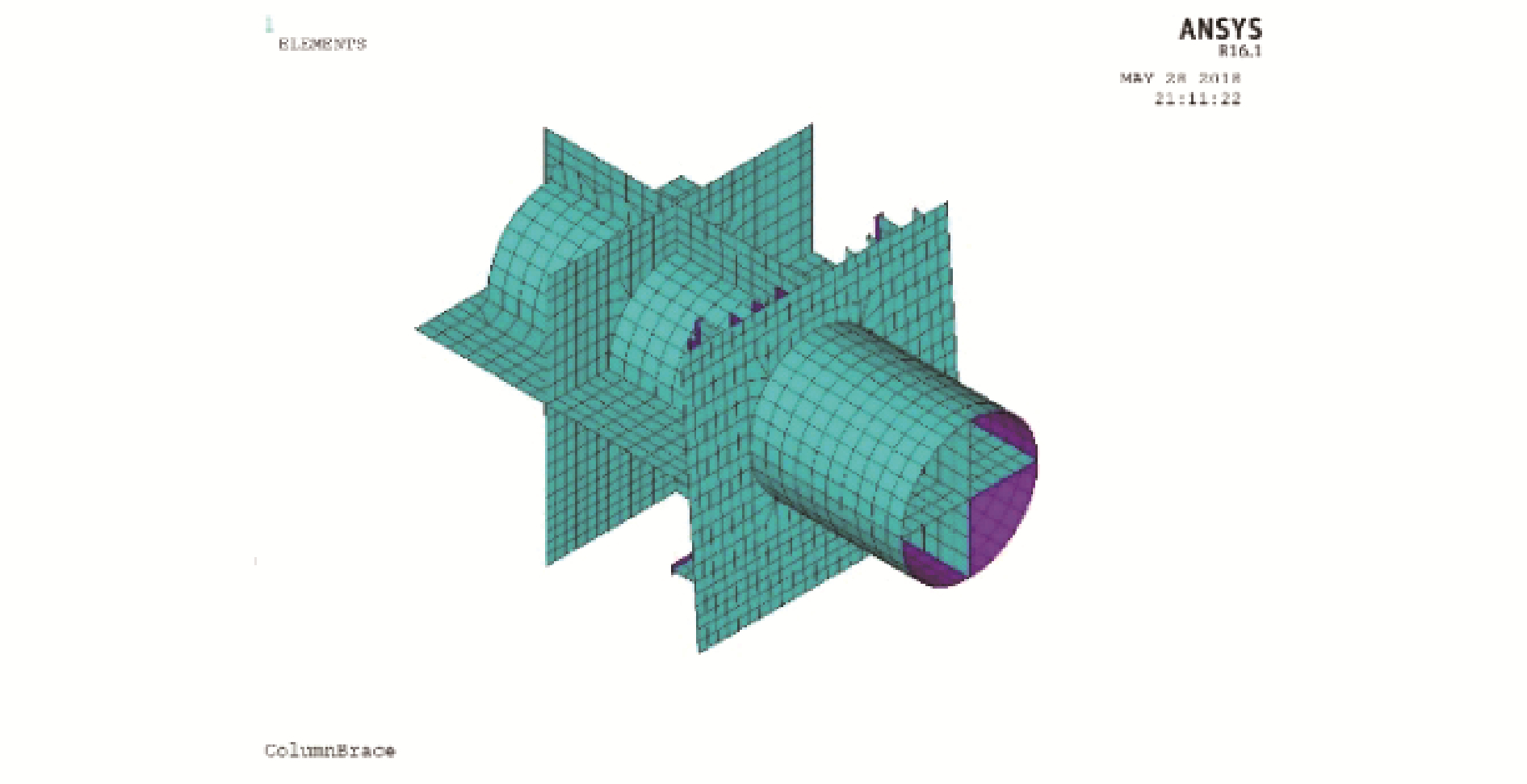Download: 图 4 局部结构有限元模型 Fig. 4 Local FE model

3.2 板厚腐蚀余量计算表 3 平台区域板厚腐蚀量与腐蚀板厚 Table 3 Plate thickness diminution and corroded thickness
3.3 结构节点应力响应分析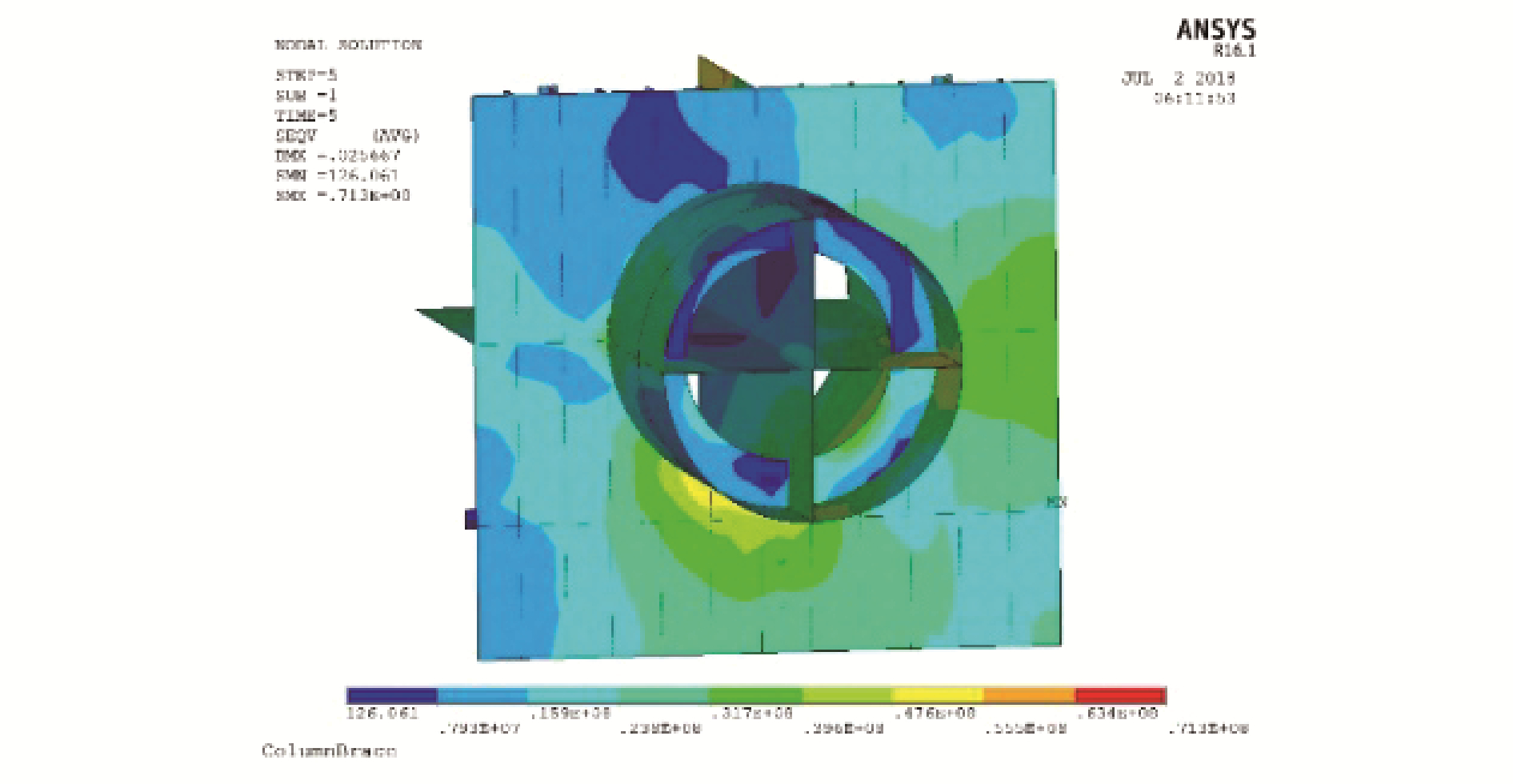Download: 图 5 Von Mises等效应力分布 Fig. 5 Von Mises stress distribution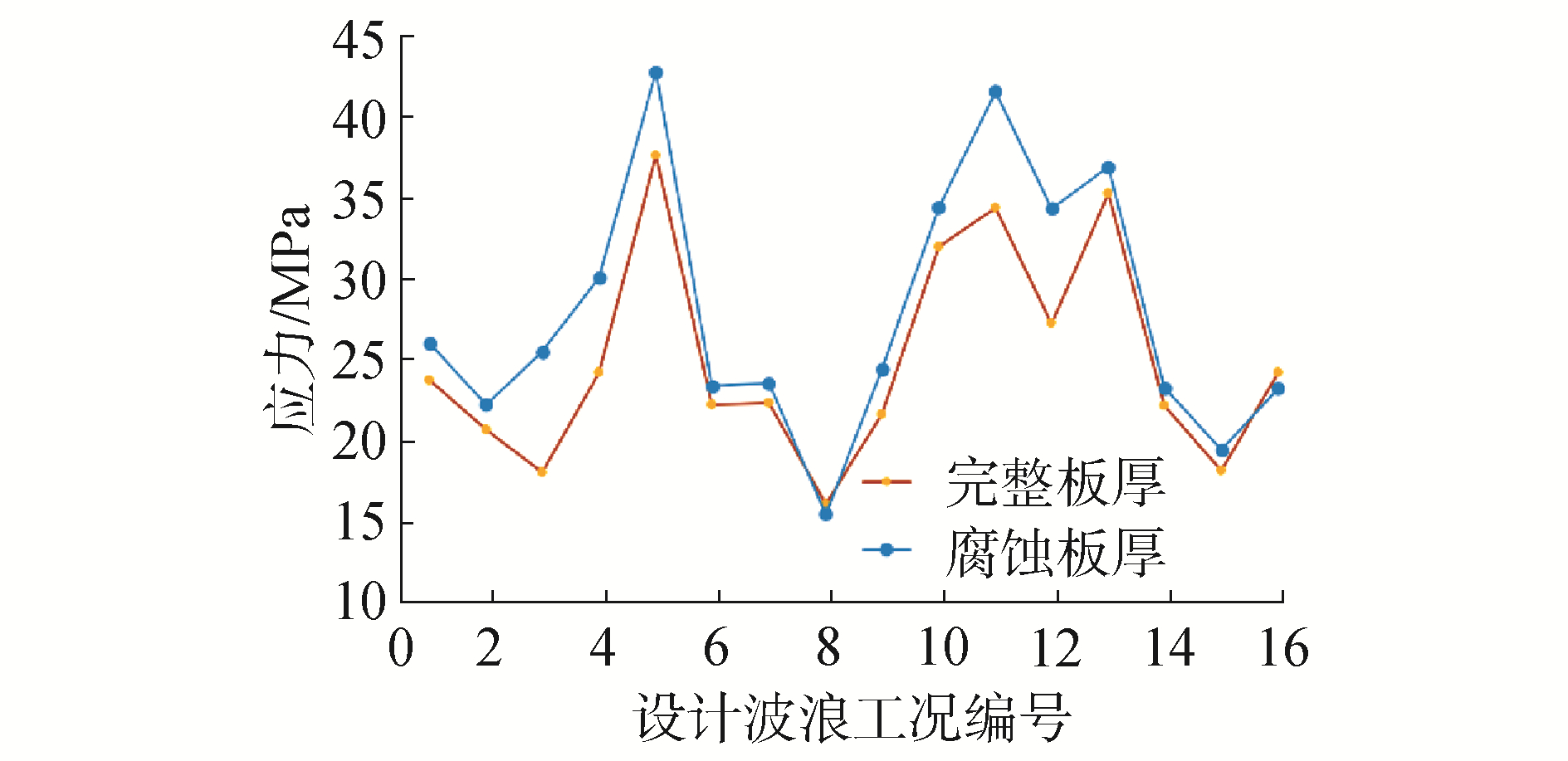Download: 图 6 2种板厚下节点应力响应对比 Fig. 6 Comparison on stresses under two plate thicknesses

3.4 疲劳裂纹扩展评估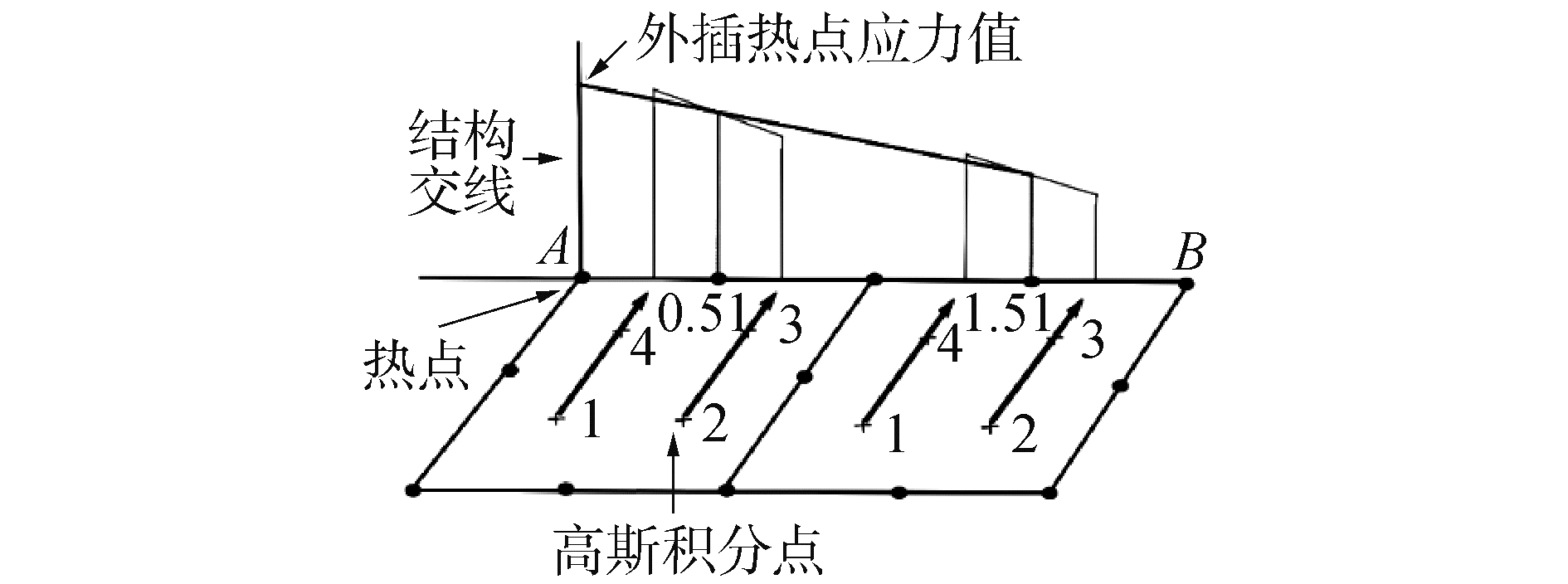Download: 图 7 线性外插热点应力示意图 Fig. 7 Hot spot stress extrapolation

 ${\sigma _{hs}} = 0.5 \times \left( {3{\sigma _{0.5t}} - {\sigma _{1.5t}}} \right)$表 4 完整板厚结构裂纹扩展临界参数 Table 4 Critical parameters under complete structure表 5 腐蚀板厚结构裂纹扩展临界参数 Table 5 Critical parameters under thickness wastage
3.5 结果分析与讨论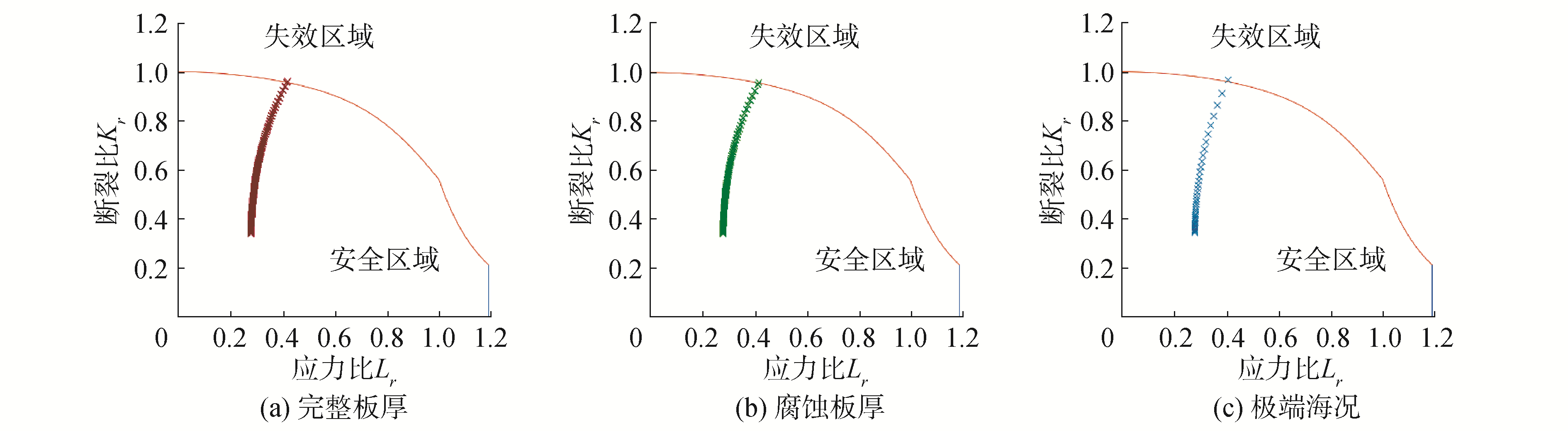Download: 图 8 疲劳裂纹扩展评估点轨迹 Fig. 8 Tracks of assessment points表 6 两种板厚下的评估点数目对比 Table 6 Comparison of assessment point counts under 2 plate thicknesses表 7 不同载荷下的疲劳裂纹扩展结果 Table 7 Fatigue crack results under 2 loadcases

4 结论

  PARIS P C, ERDOGAN F A. A critical analysis of crack propagation laws[J]. Journal of basic engineering, 1963, 85(4): 528-533. DOI:10.1115/1.3656900 (0)  LIE S T, CHIEW S P, LEE C K, et al. Fatigue performance of cracked tubular T joints under combined loads. Ⅱ:Numerical[J]. Journal of structural engineering, 2004, 130(4): 572-581. DOI:10.1061/(ASCE)0733-9445(2004)130:4(572) (0)  LIE S T, YANG Z M. Fracture assessment of damaged square hollow section (SHS) K-joint using BS7910:2005[J]. Engineering fracture mechanics, 2009, 76(9): 1303-1319. DOI:10.1016/j.engfracmech.2009.02.008 (0)  邵永波, 宋生志, 李涛. 基于失效评定图(FAD)研究含疲劳裂纹T型圆钢管节点的安全性[J]. 工程力学, 2013, 30(9): 184-193. SHAO Yongbo, SONG Shengzhi, LI Tao. Study on safety of circular tubular T-joints containing fatigue crack based on failure assessment diagram (FAD)[J]. Engineering mechanics, 2013, 30(9): 184-193. (0)  杜丽影, 邱保文, 薛欢, 等. 波浪载荷作用下海洋平台用钢焊接接头的疲劳寿命预测[J]. 焊管, 2015, 38(1): 69-72. DU Liying, QIU Baowen, XUE Huan, et al. Fatigue life prediction of the offshore platform steel welded joint under wave load[J]. Welded pipe and tube, 2015, 38(1): 69-72. DOI:10.3969/j.issn.1001-3938.2015.01.020 (0)  崔磊, 何勇, 毛江鸿, 等. 基于裂纹扩展的深水半潜式平台疲劳寿命分析[J]. 船舶力学, 2013, 17(11): 1318-1327. CUI Lei, HE Yong, MAO Jianghong, et al. Crack propagation-based fatigue life analysis for deepwater semi-submersible platform[J]. Journal of ship mechanics, 2013, 17(11): 1318-1327. DOI:10.3969/j.issn.1007-7294.2013.11.012 (0)  许亮斌, 陈国明. 考虑疲劳失效的海洋平台动态可靠性分析[J]. 石油学报, 2007, 28(3): 131-134. XU Liangbin, CHEN Guoming. Dynamic reliability analysis of offshore platform considering fatigue failure[J]. Acta petrolei sinica, 2007, 28(3): 131-134. DOI:10.3321/j.issn:0253-2697.2007.03.028 (0)  许亮斌, 陈国明. 考虑断裂和腐蚀失效的海洋平台动态可靠性研究[J]. 石油学报, 2009, 30(1): 132-135. XU Liangbin, CHEN Guoming. Evaluation method for dynamic reliability of offshore platform considering fracture and corrosion failures[J]. Acta petrolei sinica, 2009, 30(1): 132-135. DOI:10.3321/j.issn:0253-2697.2009.01.027 (0)  BSI. BS 7910, Guide to methods for assessing the acceptability of flaws in metallic structures[S]. London: British Standards Institution, 2013. (0)  黄小平, 贾贵磊, 崔维成, 等. 海洋钢结构疲劳裂纹扩展预报单一扩展率曲线模型[J]. 船舶力学, 2011, 15(1/2): 118-125. HUANG Xiaoping, JIA Guilei, CUI Weicheng, et al. Unique crack growth rate curve model for fatigue life prediction of marine steel structures[J]. Journal of ship mechanics, 2011, 15(1/2): 118-125. (0)  HUANG Xiaoping, MOAN T, CUI Weicheng. A unique crack growth rate curve method for fatigue life prediction of steel structures[J]. Ships and offshore structures, 2009, 4(2): 165-173. DOI:10.1080/17445300902732370 (0)  DNV G L. DNVGL-CG-0172, Thickness diminution for mobile offshore units[S]. Norway: Det Norske Veritas, 2015. (0)  王燕舞, 崔维成. 考虑腐蚀影响的船舶结构可靠性研究现状与展望[J]. 船舶力学, 2007, 11(2): 307-320. WANG Yanwu, CUI Weicheng. Current status and future directions for reliability analysis of ship structures under corrosion[J]. Journal of ship mechanics, 2007, 11(2): 307-320. DOI:10.3969/j.issn.1007-7294.2007.02.019 (0)  PAIK J K, WANG Ge, THAYAMBALLI A K, et al. Time-dependent risk assessment of aging ships accounting for general/pit corrosion, fatigue cracking and local denting[C]//Proceedings of the 2003 SNAME Annual Meeting in San Francisco. 2003: 219-255. (0)  GARBATOV Y, SOARES C G, WANG G. Nonlinear time dependent corrosion wastage of deck plates of ballast and cargo tanks of tankers[J]. Journal of offshore mechanics and arctic engineering, 2006, 129(1): 48-55. (0)  QIN Shengping, CUI Weicheng. Effect of corrosion models on the time-dependent reliability of steel plated elements[J]. Marine structure, 2003, 16(1): 15-34. DOI:10.1016/S0951-8339(02)00028-X (0)  Yanamoto N, Ikegami K A. Study on the degradation of coating and collision of ship's hull based on the probabilistic approach[C]//Proceedings of the International Offshore Mechanics and Arctic Engineering Symposium. 1996, 2: 159-166. (0)  DNV GL. DNV-RP-C103, Column-stabilised units[S]. Norway: DET Norske Veritas, 2005. (0)  DNV GL-RP-0005, Fatigue design of offshore steel structures[S]. Norway: DET Norske Veritas, 2014. (0)